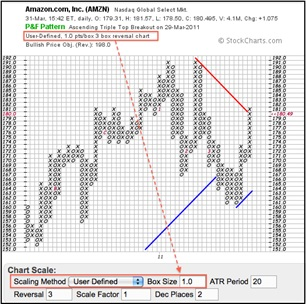# Reversal Amount

Posted in Finance, Accounting and Economics Terms, Total Reads: 839

## Definition: Reversal Amount

Reversal amount is the amount of change in price of the stock required to move the chart to the right and in the opposite direction in a Point and Figure chart. Daily movements of the price of a stock are depicted in a point & figure chart, which are represented by X & O. The chart does not have any consideration of time. It consists of a series of columns of either X’s or O’s. In the tabular representation, the X's & O's present in the columns depict rising and falling prices respectively.

There are two variables that are required to plot a point and figure chart, one is the box size and the other is the reversal amount. Box size is the minimum amount that the price must move in order to plot a new X. Reversal amount determines the minimum amount that the price needs to move in the opposite direction for a column reversal to take place. Whenever the reversal amount is crossed, a new column is started to the right with X’s or O’s, moving in the opposite direction.

Example: Daily high and low prices of a stock are given.

 High Low Day 1 15.50 12.90 Day 2 12.60 10.90 Day 3 14.10 11.95 Day 4 15.99 13.80 Day 5 15.10 12.00

The box size is 1 and reversal amount is 3. Below is the procedure to plot a Point and Figure chart.

Day 1: Since the day’s high is 15.50 and low is 12.90, we assume that the price is falling and hence plot O’s in the boxes 15, 14 & 13

 16 15 O 14 O 13 O 12 11 10

Day 2: The day’s high is 12.60 and low is 10.90. Since the current column is an O column, we need to check whether the day’s low is significant enough to plot another O. As 10.90 < 12.90, we can plot 2 more O’s and hence we ignore the high.

 16 15 O 14 O 13 O 12 O 11 O 10

Day 3: The day’s high is 14.10 and low is 11.95. Since we are in an O column, we need to check the day’s low (11.95). As 11.95 is greater than the current low 10.90, we cannot plot a new O. Next we need to see whether the high is greater than or equal to the current value plus the reversal amount (3). Since the high 14.10 is greater than 11+3= 14, we need to add a new column with 3 X’s.

 16 15 O 14 O X 13 O X 12 O X 11 O 10

Day 4: The day’s high is 15.99 and low is 13.80. The current column is a X column, we need to check whether the day’s high is greater than the current price. Since 15.99 > 14.10, and since the difference is greater than the box size (1), we need to draw a new X and the low is ignored.

 16 15 O X 14 O X 13 O X 12 O X 11 O 10

Day 5: The day’s high is 15.10 and low is 12.00. The current column is a X column, we need to check the day’s high, since 15.10 < 15.99, we cannot plot a new X. Next we need to check whether the low is less than or equal to the current value minus the reversal amount. Since 12.00 is less than 15.99-3.00= 12.99, we need to plot 3 O’s in the next column.

 16 15 O X 14 O X O 13 O X O 12 O X O 11 O 10Source: stockcharts

A sample Point and Figure chart is shown above

 High Low Day 1 15.50 12.90 Day 2 12.60 10.90 Day 3 14.10 11.95 Day 4 15.99 13.80 Day 5 15.10 12.00

Hence, this concludes the definition of Reversal Amount along with its overview.

Browse the definition and meaning of more terms similar to Reversal Amount. The Management Dictionary covers over 7000 business concepts from 6 categories. This definition and concept has been researched & authored by our Business Concepts Team members.

Search & Explore : Management Dictionary

Similar Definitions from same Category: